# Approximate non-conformal potential

Jump to: navigation, search

The Approximate non-conformal potential (ANC) is given by (Eq 2.16 in ):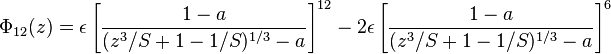$\Phi_{12}(z) = \epsilon \left[ \frac{1-a}{(z^3/S +1 - 1/S)^{1/3} -a} \right]^{12} - 2\epsilon \left[ \frac{1-a}{(z^3/S +1 - 1/S)^{1/3} -a} \right]^{6}$

where

•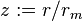$z := r/r_m$
•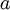$a$ is the hard-core diameter in units of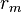$r_m$
•$\epsilon$ is the well depth
•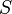$S$ is a softness parameter

## Second virial coefficient

The second virial coefficient is given in .